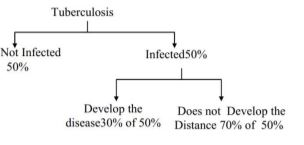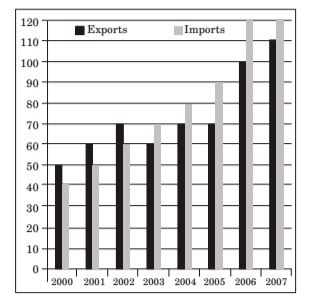## Numerical Ability

#### Numerical Ability

1. A person moving through a tuberculosis prone zone has a 50% probability of becoming infected. However, only 30% of infected people develop the disease. What percentage of people moving through a tuberculosis prone zone remains infected but does not show symptoms of disease?
1. 15
2. 33
3. 35
4. 37

1.= 70 × 50 ⇒ 35 = 35% 100 100 100

∴ Option (c) is correct

##### Correct Option: C= 70 × 50 ⇒ 35 = 35% 100 100 100

∴ Option (c) is correct

1. The exports and imports (in crores of Rs) of a country from 2000 to 2007 are given in the following bar chart. If the trade deficit is defined as excess of imports over exports, in which year is the trade deficit 1/5th of the exports?1. 2005
2. 2004
3. 2007
4. 2006

1. For 2005, trade defict = (90 – 70) crores = 20 crores

 Now , 1th of export = 1 (70) crores = 14 crores ≠ 20 crores. 5 5

Hence option (a) is wrong.
For 2004, Trade defict = (80 – 70) crores = 10 crores
 Now , 1th of export = 1 (70) crores = 14 crores ≠ 20 crores. 5 5

Hence option (b) is wrong.
For 2007, Trade defict = (120 – 110) crores = 10 crores
 Now , 1th of export = 1 (110) crores = 22 crores ≠ 10 crores. 5 5

Hence option (c) is wrong.
For 2006, Trade defict = (120 – 100) crores = 20 crores
 Now , 1th of export = 1 (100) crores = 20 crores = Trade deficts 5 5

Hence option (d) is correct.

##### Correct Option: D

For 2005, trade defict = (90 – 70) crores = 20 crores

 Now , 1th of export = 1 (70) crores = 14 crores ≠ 20 crores. 5 5

Hence option (a) is wrong.
For 2004, Trade defict = (80 – 70) crores = 10 crores
 Now , 1th of export = 1 (70) crores = 14 crores ≠ 20 crores. 5 5

Hence option (b) is wrong.
For 2007, Trade defict = (120 – 110) crores = 10 crores
 Now , 1th of export = 1 (110) crores = 22 crores ≠ 10 crores. 5 5

Hence option (c) is wrong.
For 2006, Trade defict = (120 – 100) crores = 20 crores
 Now , 1th of export = 1 (100) crores = 20 crores = Trade deficts 5 5

Hence option (d) is correct.